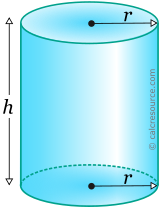## Properties of Cylinder

This tool calculates the basic geometric properties of a cylinder, with a circular base. Enter the shape dimensions 'r' and 'h' below. The calculated results will have the same units as your input. Please use consistent units for any input.

 r = h =
 Geometric properties: Volume = Surface area = Base area = Lateral surface area =## Definitions

### Geometry

The cylinder is a solid object enclosed by two parallel planar bases and a lateral cylindrical surface. Typically the most recognizable cylinder is the one with circular bases.

The volume of a circular cylinder is given by the formula:

where r the radius of the circular base and h the height of the cylinder.

The lateral surface area of the cylinder, can be found using the following formula:

Therefore the total surface area, including both bases and the lateral surface is found as:

where the base area.